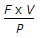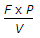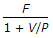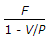# Mechanical Engineering - Industrial Engineering and Production Management

Exercise : Industrial Engineering and Production Management - Section 2
46.
If F is the fixed cost, V is the variable cost per unit (or total variable costs) and P is the selling price of each unit (or total sales value), then break even point is equal toExplanation:
No answer description is available. Let's discuss.

47.
CPM stands for
Combined Process Method
Critical Path Method
Common Planning Method
Critical Process Method
Explanation:
No answer description is available. Let's discuss.

48.
The average time recorded by work study man for an operation is called
standard time
normal time
representative time
none of these
Explanation:
No answer description is available. Let's discuss.

49.
In value engineering, important consideration is given to
customer satisfaction
function concept
profit maximisation
cost reduction